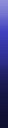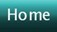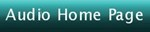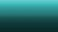Created January 2, 2010
Updated January 27, 2010.  See document history at end for details.

## JFET Phono Preamp

This is an example only.  It is not intended to be final or complete design.

Figure 1:  Schematic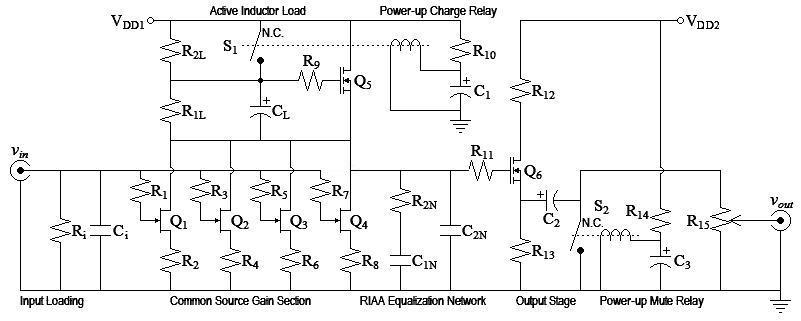Parts List Q1-Q4 2N3918 n-ch jfet Q5,Q6 BS170 n-ch mosfet R1,R3,R5,R7 220Ω, 5% R2,R4,R6,R8 220Ω, 5% R2L 165kΩ, 1% R2N 23.7kΩ, 1% C1N,a 13nF, 1% or 5% C1N,b 240pF, 1% or 5% C2N,a 4.3nF, 1% or 5%
 C2N,b 240pF, 1% or 5% R1L 105kΩ, 1% CL 10,000µF, 25V electrolytic R9,R11 1kΩ, 5% R12 82Ω, 5% R13 1kΩ, 5% C2 100µF, 25V electrolytic R15 1kΩ pot S1,S2 SPST reed relay, normally closed, coil rated 10mA@5V
Remaining values are left for you to calculate.  See text.

#### Initial Design Decisions

• Discrete voltage input design with 46db gain at 1kHz will amplify 5mV rms input to produce 1V rms before attenuator.
• Q1-Q4 are 2N3918 n-ch jfet (6nV/rtHz noise is not bad, you may choose a better device)
• I chose four devices because multiple parallel devices increase S/N by sqrt(# of devices).  In this case the effective noise specification is bettered to 3nV/rtHz.
• Q5,Q6 are BS170 n-ch mosfet (commonly available)
• vgs,max of Q6 of 20V limits VDD due to startup relays.  Choose VDD = 18V.
• Let bias of active inductor set bias to gate of Q6 to eliminate coupling capacitor.
 vg,Q5 = (VDD-vgs-Q5-max)+vsg-Q1-max 2 = 18V-2V+8V 2 = 12V
• R2L of active inductor functions as R1 of passive RIAA network.
• I chose to do the small signal class-a design with a fourier series based distortion predictor tool out of convenience.  Small signal class-a design is not in the scope of this article.
• Because of possibility of negative feedback from buffer stage back to gain stage through power supply, I decided to recommend two power supply taps.

#### Gain Stage Design

Although I used a convenient fourier series analysis tool to do the small signal analysis, there were some design decisions.  I wanted to choose gate and source resistors small enough to make their noise contribution insignificant compared to the JFET.  This would lower noise and allow the possiblity of noise improvement with better JFETs.
 Resistor noise equation (per rtHz, figure of merit, does not account for bandwidth) vn-resistor-rtHz = sqrt(4kTR)
Rearrange resistor noise equation to calculate equivalent input noise resistance of JFET.
 R = vn-resistor-rtHz2 4kT = 6nV2 4 x 1.3806504e-23 x 298.15ºK = 2.186371576kΩ
I choose RG and RS 10 times lower to make their noise contribution insignificant.
R1-R8 = 220Ω.
Because the fourier series analysis resulting from this choice was excellent, I made no further adjustments to these values.

#### Fourier Series Analysis of Q1-Q4 (excluding drain load)

Analysis of Common Source Circuit
transistor type = JFET
transconductance = 5.5mS
threshold voltage = -3V
source resistor = 220Ω

Input signal (peak) = 7.0710678mV
Input bias = 0V
Output signal (peak) = 21.585572µA
Output bias = 7.8662828mA1
Gain = 3.0526609mS

Total distortion = 1.6210607nA = 0.00750993% = -82.4873dB

Breakdown by harmonic

 harmonic value percent dB 0 7.8662844mA1 36442.32534044% 51.23dB DC Offset 1 21.585572µA 100.00000000% 0.00dB Fundamental 2 1.6210607nA 0.00750993% -82.49dB

#### RIAA Analysis

Refer Calculating Passive RIAA Equalization in article Phono Equalization Calculations.

From this fourier analysis we can calculate R1 of Passive RIAA network (aka R2L)
 R2L = (Low frequency RIAA boost relative to 1kHz) x (1kHz output voltage) (Output signal current) x (#parallel devices) = 10 x 1.414213562V 21.585572µA x 4 = 163.791532kΩ
Round to nearest 1% value.  R2L = 165kΩ

Given R1:R2 = 6.877358491
 R2N = 165kΩ 6.877358491 = 23.99176955kΩ
Round to nearest 1% value.  R2N = 23.7kΩ

Given R1C1 = 2187µS
 C1N = 2187µS 165kΩ = 13.25454545nF
Round down to nearest 5% value: 13nF
Choose parallel 5% value to makeup remainder:  240pF
C1N = 13nF + 240pF

Given R1C2 = 750µS
 C2N = 750µS 165kΩ = 4.545454545nF
Round down to nearest 5% value: 4.3nF
Choose parallel 5% value to makeup remainder:  240pF
C2N = 4.3nF + 240pF

#### Active Inductor Analysis

• Choose R2L for gain of overall circuit.
R2L chosen already in RIAA analysis.
BS170 specification:  gm = 320mS.
gfs = 2 x sqrt(gmiD) = 2 x sqrt(320mS x 7.8662828mA x 4) = 200.6872391mS
R1L =

R2L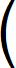Vbias vT+sqrt(iD/gm) -1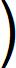=
165kΩ6V 2V+sqrt((7.8662828mA x 4)/320mS) -1= 103.5527796kΩ
Round to nearest 1% value:  R1L = 105kΩ
• Choose CL to set the pole frequency the desired amount below the passband.  (equation (21))
 CL = gfsR1L+1 2πfPOLER1L = (200.6872391mS x 105kΩ)+1 2π x 5Hz x 105kΩ = 6.388376376mF
6800µF would work here.  I would rather choose a larger common value (CL = 10,000µF) and solve for a lower pole.
 fPOLE = gfsR1L+1 2πCLR1L = (200.6872391mS x 105kΩ)+1 2π x 10mF x 105kΩ = 3.194188188Hz
• Determine if a bypass switch is needed to charge the capacitor on startup.
Power on relay added at beginning of design.
Calculate charge time for CL:
 i = C δv δt
Rearrange capacitor equation above and calculate time.  (2V presumes vgs not much more than vT)
 Δt = CΔv iD = 10mF x 2V 7.8662828mA x 4 = 635.6242366mSec

#### Q6 Circuit Design

My design goal here was reasonably low output impedance.  1kΩ output impedance would have been low enough to minimize voltage loss into a typical 47kΩ load.  I choose 500Ω instead on the far chance of driving a 600Ω load with a tolerable voltage loss.

#### Fourier Series Analysis of Q6 Circuit

Note:  This analysis based on a more convenient equivalent model of source circuit.  Two 500Ω are modeled in series with one bypassed by a capacitor in place of the parallel circuit shown.
Analysis of Common Source Circuit
transistor type = MOSFET
transconductance = 320mS
threshold voltage = 2V
source resistor = 500Ω

Input signal (peak) = 1.4142136V
Input bias = 7V
Output signal (peak) = 2.7910912mA
Output bias = 9.8041739mA1
Gain = 1.9735995mS

Total distortion = 950.79116nA = 0.03406521% = -69.3538dB

Breakdown by harmonic

 harmonic value percent dB 0 9.805058mA1 351.29836800% 10.91dB DC Offset 1 2.7910912mA 100.00000000% 0.00dB Fundamental 2 888.84698nA 0.03184586% -69.94dB 3 56.727241nA 0.00203244% -93.84dB 4 4.7600566nA 0.00017054% -115.36dB 5 456.88194pA 0.00001637% -135.72dB

#### Miscellaneous Calculations

R12 limits charge current through Q6 to C2 to <500mA
R12 >= 20V/500mA = 40Ω
Choose R12 = 82Ω.

Want to set C2 for as low a highpass pole as CL.  First find Requiv of pole.  Output impedance of Q6 is the inverse of its operating ac transconductance gfs.
gfs = 2 x sqrt(gmiD) therefore
 Rout-Q6 = 1 2 x sqrt(gmiD) = 1 2 x sqrt(320mS x 9.8041739mA) = 8.92667066Ω

 Requiv = (Rout-Q6 || R13) + R15 = Rout-Q6 x R13 Rout-Q6 + R13 + R15 = 1.00884769kΩ

 C2 = 1 2πfpoleRequiv = 1 2π x 2.348779238Hz x 1.00884769kΩ = 67.16643973µF
Choose C2 = 100µF.

Choose R10 and  R14 to set 10mA through relay coil.
 R10 = VDD-vCOIL iCOIL = 18V-5V 10mA = 1.3kΩ

Calculate load current from power supplies VDD1 and VDD2.
iDD1 = iQ1-BIAS x 4 + iRELAYCOIL = 7.8662828mA x 4 + 10mA  = 41.4651312mA
iDD2 = iQ6-BIAS + iRELAYCOIL = 9.8041739mA + 10mA = 19.8041739mA

#### Design Decisions Left to You

• Ri and Ci should be calculated per article Phono Termination Calculations and Calculator.
• Gate resistors were somewhat arbitrarily chosen.  They seem to be good values.  I know that the 1kΩ values work with the BS170.  I have seen many jfet schematics without gate resistors at all.  Increase them if any oscillations occur.
• Relay components C1 and C3 must be calculated as follows:
C1 must be chosen by calculation or experimentation to exceed CL charge time calculated above (432.2244809mSec).  C3 would then be chosen for a somewhat longer delay to prevent a pop from the activation of the other relay.
• This circuit and its analysis is only given as an example; change everything if you like.
• A low noise 18V power supply.  I recommend the circuit of article Line Level Class A Power Supply.

1There is a seeming discrepancy in the fourier analysis between the cited output bias and the same value given in the analysis.  The first value is the bias without any signal and the second with a signal. This effect is created by the same asymetrical transfer curve that gives us a desireable second order distortion characteristic.  The positive half of the signal is amplified more than the negative half.  As a result the dc bias is modulated by the average signal level. This small component amounts to AM demodulation of the  music signal.  Abstractly, I had already anticipated this effect.  I would be curious what effect this has on the overall sound.  Is is detrimental, or does it add an extra euphonic bass urge?

Document History
January 2, 2010  Created